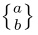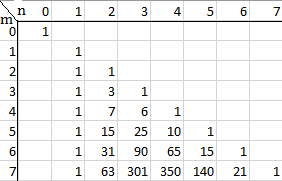# Stirling Numbers of the Second Kind

In combinatorics, the Stirling numbers of the second kind tell us how many ways there are of dividing up a set of n objects (all different, or at least all labeled) into k nonempty subsets. The k subsets aren’t labeled.

We write Stirling numbers of the second kind as S(n,k) or:.

## Examples of Stirling Numbers of the Second Kind

What is S(3,3)? This question asks “How many ways can we partition a set of three elements into three subsets?” A set of three elements — say, the set {0,1,2} — can be partitioned into three subsets exactly one way: {1,2,3}, so S(3,3) is just 1. In fact, since a set of k elements can always be partitioned into a set of k nonempty subsets exactly one way, S(n,n) is always 1.

S(3,2) will be the number of ways we can partition our set of three elements into two subsets. There are three possible ways to do this; each splits the set into two pieces made up of one element and two elements. Using our example set of {0,1,2}, we can come up with:
{{0,1}{2}}
{{0}{1,2}}
{{1}{0,2}}.
So S(3,2)= 3.

S(3,1) is the number of ways we can partition our elements into just one subset; since the only possibility of that is the set including every element, {0,1,2}, S(3,1) is also 1. This can also be generalized; S(n,1) = 1 for every n.

The following table (from Quaintance, 2015) shows the first few possibilities for Stirling numbers of the second kind:## Calculating Stirling Numbers of the Second Kind

There are two ways of calculating Stirling numbers of the second kind. First,they can be calculated recursively; i.e, with reference to lower order Stirling numbers of the second kind.

S(m,n) = S(m – 1,n – 1) + nS(m – 1,n).
Where:

• m is the number of elements in the original set,
• n is the number of subsets.

This simple formula makes it easy to make tables of Stirling numbers of the second kind, or to find a number when we know the figures for smaller sets.

We saw above that S(3,2) = 3 and S(3,1) = 1. We can use these two facts to enable us to find S(4,2), since with m = 4 and 2 = n, the formula above becomes:
S(4,2) = S(3,1) + 2 * S(3,2) = 1 + 2 * 3 = 7.

There’s also a formula that can be used to calculate S(n,k) for any n or k. This is given byHere ! means a factorial, and the large Σ means that what comes after it is summed up from i = 0 to n.

## References

Quaintance, J. (2015). Combinatorial Identities for Stirling Numbers: The Unpublished Notes of H W Gould. World Scientific, Oct 27.

CITE THIS AS:
Stephanie Glen. "Stirling Numbers of the Second Kind" From StatisticsHowTo.com: Elementary Statistics for the rest of us! https://www.statisticshowto.com/stirling-numbers-second-kind/